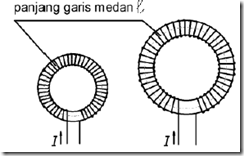" /> Magnetic Field Around Coil | TN Elektro
Home > English > Magnetic Field Around Coil

# Magnetic Field Around Coil

A direct current flows through the coil, then a magnet is brought closer needle around the coil. Turns out the coil has properties like a bar magnet, with a north poles and the south, as shown in Figure 1114.Image 1114 Determination of the poles in the coil is energized.

The current flow in the coil should play a screw. In Figure 1.114a visible screw rotates to the right, the screw will be moved forward. At the direction of forward motion screw will be the north pole (U) and the other becomes the south pole (S). In the right image shows how the north pole south coils can terbangkit. The right image visible pieces of the coil, which look half coil on the bottom, so that the conductor cross-section visible from above.

So on the bottom side of the current is left so that it is crossed, while the top of the current comes to us so marked point. In the conductor cross-section, with the right-hand rule, the direction of the magnetic field is turned to the right. Due to the current direction penhantar beside the same, then the magnetic field would annihilate them. Meanwhile, if the cross-section between the upper and lower, the current in opposite directions, then the direction of the field Salang strengthen them so that this conductor diatara field direction will have a direction to the right.

Then the right side of the coil will have a north pole (U) and the left side will have the south pole (S). In Figure 1.114b with reverse current flow, hence the poles turned around. The right side of the south pole (S) and the left side of the north pole (U). Hanging Magnet massive piece of iron so with a measure of power, so that the iron can get into containers with wire coils with 600 coil email. Coil rated voltage supply, up to 2A current flows.

Make a note of the appointment of measuring power. Then replace the containers with the coil with 1200 the number of coils. Supply voltage is changed so that the current flowing at 1A. From the experiments above, was a powerful force shown the same magnitude. From the experiments shown the relationship between current and many coils and power. This relationship can be written with the following formula:
Θ = I. N ……………………………… (1,109)
Θ = GGM / emf (force magnet / elektromotive force)
I = current strength
N = Number of coils

Number coil has no units that Θ have the same unit with strong arus.Tetapi times the amount in the form of Amper Lilit. Example: a coil 6000. The coil and is energized by 0,1A, how much Θ of the coil?
Answer: Θ = I. N = 0.1. 6000
At the core of the coil with a ring as 1115 Great Image has a long field lines larger than the small coil. To generate the magnetic field in the coil is large with the same field strength required greater energy than the generation on a small coil.Image 1,115 small and large coils